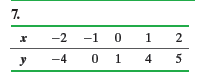×
Get Full Access to Statistics: Informed Decisions Using Data - 5 Edition - Chapter 14.1 - Problem 7
Get Full Access to Statistics: Informed Decisions Using Data - 5 Edition - Chapter 14.1 - Problem 7

×

# ?In Problems 5–10, use the results of Problems 7–12, respectively, from Section 4.2 to answer the following questions: (a) What are the estimaISBN: 9780134133539 240

## Solution for problem 7 Chapter 14.1

Statistics: Informed Decisions Using Data | 5th Edition

• Textbook Solutions
• 2901 Step-by-step solutions solved by professors and subject experts
• Get 24/7 help from StudySoup virtual teaching assistantsStatistics: Informed Decisions Using Data | 5th Edition

4 5 1 309 Reviews
23
3
Problem 7

In Problems 5–10, use the results of Problems 7–12, respectively, from Section 4.2 to answer the following questions:

(a) What are the estimates of b0 and b1?

(b) Compute the standard error, the point estimate for s.

(c) Determine sb1.

(d) Assuming the residuals are normally distributed, test H0: b1 = 0 versus H1: b1 ≠ 0 at the a = 0.05 level of significance.Step-by-Step Solution:

Step 1 of 5) The results of Problems 7–12, respectively, from Section 4.2 to answer the following questions: (a) What are the estimates of b0 and b1 (b) Compute the standard error, the point estimate for s. (c) Determine sb1. (d) Assuming the residuals are normally distributed, test H0: b1 = 0 versus H1: b1 ≠ 0 at the a = 0.05 level of significance. To clarify the roles of l and t, revisit Example 1. Here, l = 2 cars per minute, while t = 20 minutes (the length of time between 12:20 p.m. and 12:40 p.m.). Computing Probabilities of a Poisson Process Problem A McDonald’s manager knows that cars arrive at the drive-through at the average rate of two cars per minute between the hours of 12 noon and 1:00 p.m. Determine and interpret the probability of the following events: (a) Exactly six cars arrive between 12 noon and 12:05 p.m. (b) Fewer than six cars arrive between 12 noon and 12:05 p.m. (c) At least six cars arrive between 12 noon and 12:05 p.m.

Step 2 of 2

##### ISBN: 9780134133539

Statistics: Informed Decisions Using Data was written by and is associated to the ISBN: 9780134133539. The full step-by-step solution to problem: 7 from chapter: 14.1 was answered by , our top Statistics solution expert on 01/15/18, 03:19PM. This full solution covers the following key subjects: . This expansive textbook survival guide covers 88 chapters, and 2422 solutions. The answer to “?In 5–10, use the results of 7–12, respectively, from Section 4.2 to answer the following questions:(a) What are the estimates of b0 and b1?(b) Compute the standard error, the point estimate for s.(c) Determine sb1.(d) Assuming the residuals are normally distributed, test H0: b1 = 0 versus H1: b1 ? 0 at the a = 0.05 level of significance.” is broken down into a number of easy to follow steps, and 59 words. This textbook survival guide was created for the textbook: Statistics: Informed Decisions Using Data, edition: 5. Since the solution to 7 from 14.1 chapter was answered, more than 215 students have viewed the full step-by-step answer.

## Discover and learn what students are asking

Calculus: Early Transcendental Functions : Riemann Sums and Definite Integrals
?In Exercises 3–8, evaluate the definite integral by the limit definition. $$\int_{2}^{6} 8 d x$$

Calculus: Early Transcendental Functions : Slope Fields and Eulers Method
?In Exercises 1-8, verify the solution of the differential equation. Solution D

Calculus: Early Transcendental Functions : Space Coordinates and Vectors in Space
?In Exercises 1-4, plot the points in the same three-dimensional coordinate system. (a) (0, 4, -5) (b) (4, 0, 5

Unlock Textbook Solution